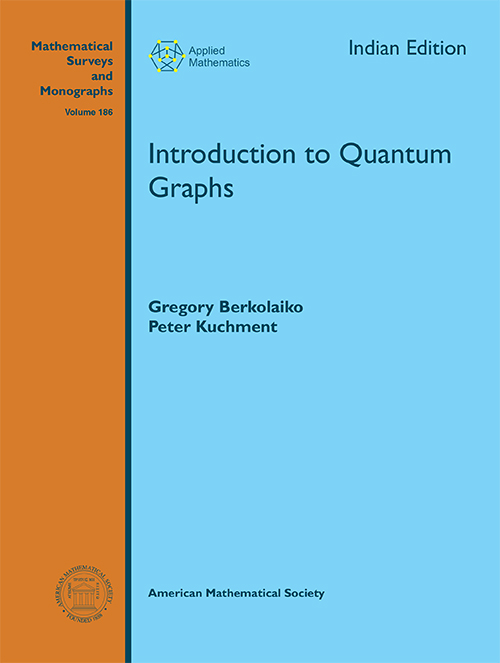## Introduction to Quantum Graphs

#### Price: 960.00

A “quantum graph” is a graph considered as a one-dimensional complex and equipped with a differential operator (“Hamiltonian”). Quantum graphs arise naturally as simplified models in mathematics, physics, chemistry, and engineering when one considers propagation of waves of various nature through a quasi-one-dimensional (e.g., “meso-” or “nano-scale”) system that looks like a thin neighborhood of a graph. Since at least the 1930S, quantum graphs techniques have been applied successfully in various areas of mathematical physics, mathematics in general and its applications. This book provides a comprehensive introduction to the topic, collecting the main notions and techniques. It also contains a survey of the current state of the quantum graph research and applications<

#### Contributors (Author(s), Editor(s), Translator(s), Illustrator(s) etc.)

Gregory Berkolaiko is Professor at the Department of Mathematics, Texas A&M University, College Station, USA.

Peter Kuchment is University Distinguished Professor at the Department of Mathematics, Texas A&M University, College Station, USA.

Preface
Introduction
Chapter 1. Operators on Graphs
Chapter 2. Quantum Graph Operators
Chapter 3. Spectra of Quantum Graphs
Chapter 4. Spectra of Periodic Graphs
Chapter 5. Spectra of Quantum Graphs
Chapter 6. Quantum Chaos on Graphs
Chapter 7. Some Applications and Generalizations
Appendix A. Some Notions of Graph Theory
Appendix B. Linear Operators and Operator-Functions
Appendix C. Structure of Spectra
Appendix D. Symplectic Geometry and Extension Theory
Bibliography
Index

`